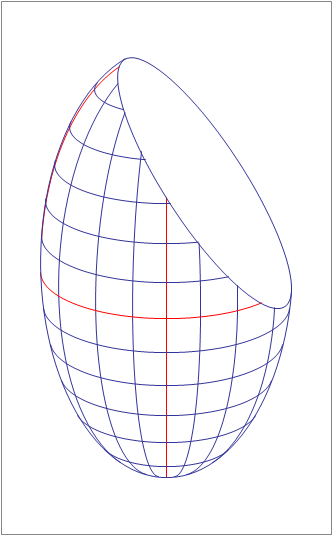# A Planar Cut Through an EllipsoidAn ellipsoid with center $(0,0,0)$ and semi-axes of lengths 10, 15, and 30 along the $x, y,$ and $z$ directions, respectively, is cut by a plane whose equation is $x + 3y + 2z = 40$.

The intersection of the ellipsoid and the plane is an ellipse.

Find its area.

This problem is original.

×